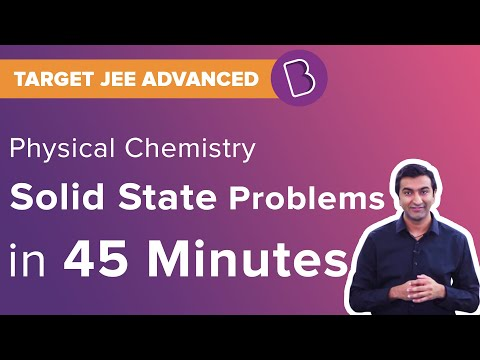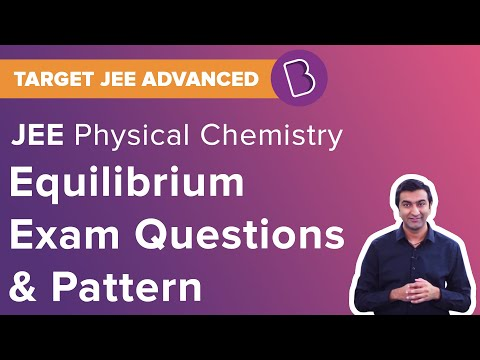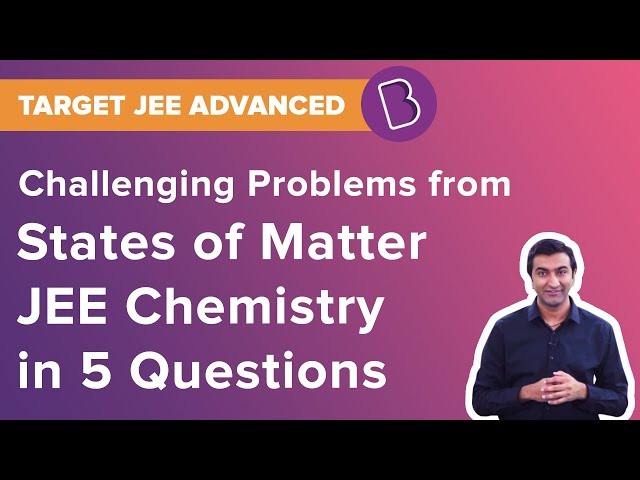Win up to 100% scholarship on Aakash BYJU'S JEE/NEET courses with ABNAT Win up to 100% scholarship on Aakash BYJU'S JEE/NEET courses with ABNAT

# JEE Main 2020 Chemistry Paper With Solutions Sept 3 (Shift 1)

JEE Main 2020 Paper with Solutions Chemistry – Shift 1 will help the students get an idea about the exam pattern, frequently asked concepts, section-wise distribution of marks and more. Practising these questions will help the candidates in increasing their solving speed along with maintaining accuracy. By solving the question papers, rigorous mistakes can be avoided. It is highly recommended to download JEE Main Sample Question Papers and solve them regularly to score high marks.
September 3 Shift 1 – Chemistry
Question 1: It is true that:
1) A second order reaction is always a multistep reaction
2) A first order reaction is always a single step reaction
3) A zero order reaction is a multistep reaction
4) A zero order reaction is a single step reaction

Factual

Solution: (3)

Question 2: An acidic buffer is obtained on mixing:
1) 100 mL of 0.1 M HCl and 200 mL of 0.1 M CH3COONa
2) 100 mL of 0.1 M HCl and 200 mL of 0.1 M NaCl
3) 100 mL of 0.1 M CH3 COOH and 100 mL of 0.1 M NaOH
4) 100 mL of 0.1 M CH3COOH and 200 mL of 0.1 M NaOHSolution: (1)

Question 3: The Kjeldahl method of Nitrogen estimation fails for which of the following reaction products?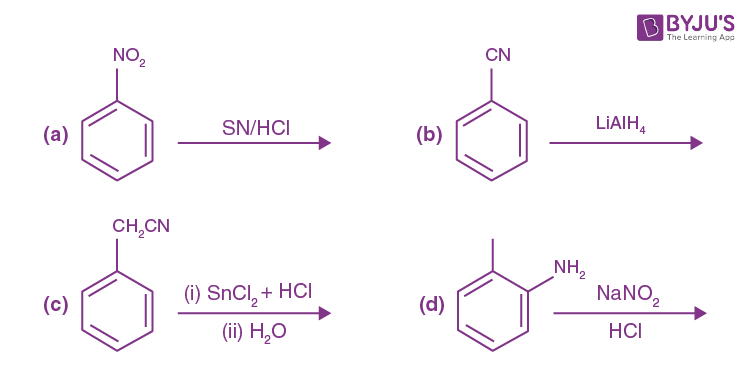1) (a), (c) and (d)
2) (b) and (c)
3) (c) and (d)
4) (a) and (d)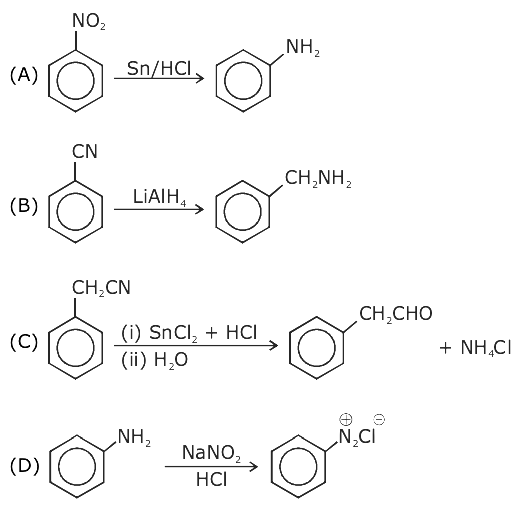Diazo compounds and inorganic nitrogen can’t be estimated by the kjeldahl method.

Solution: (3)

Question 4: If the boiling point of H2O is 373 K, the boiling point of H2S will be :
1) greater than 300 K but less than 373 K
2) equal to 373 K
3) more than 373 K
4) less than 300 K

Less than 300 K (factual)

Solution: (4)

Question 5: The complex that can show optical activity is :
1) cis– [CrCl2 (ox)2]3- (ox – oxalate)
2) trans – [Fe (NH3)2 (CN)4]
3) trans – [Cr (Cl2) (ox)2]3-
4) cis– [Fe (NH3)2 (CN)4]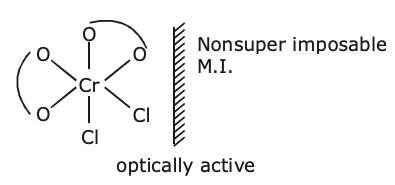cis– [CrCl2 (ox)2]3- (ox – oxalate)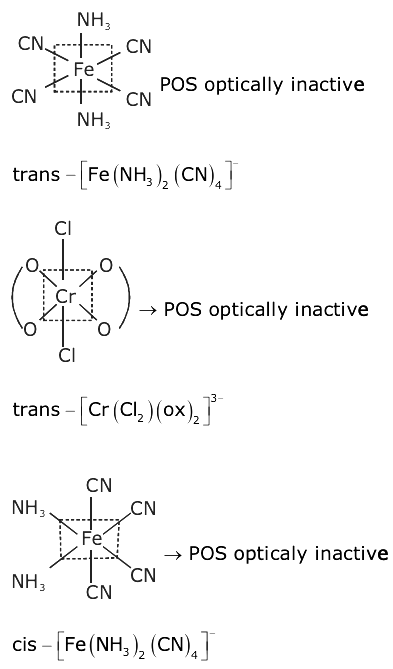Solution: (1)

Question 6: Which one of the following compounds possesses the most acidic hydrogen?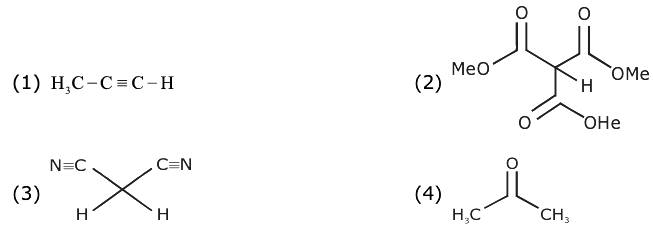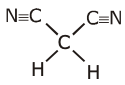has most acidic hydrogen among given compounds , this is due to the strong – M effect of –CN group which stabilize –ve charge significantly.

Solution: (3)

Question 7: Aqua regia is used for dissolving noble metals (Au, Pt, etc.). The gas evolved in this process is :
1) N2O3
2) N2
3) N2O5
4) NO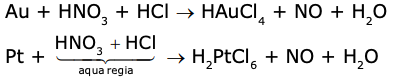Solution: (4)

Question 8: The antifertility drug “Novestrol” can react with:
1) Br2 / water; ZnCl2 / HCl; FeCl3
2) Br2 / water; ZnCl2 / HCl; NaOCl
3) Alcoholic HCN; NaOCl; ZnCl2 / HCl
4) ZnCl2 / HCl; FeCl3; Alcoholic HCN

Novestrol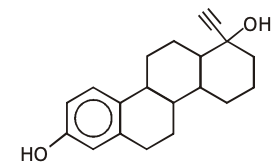It can react with Br2 / water due to presence of unsaturation, with ZnCl2 / HCl due to – OH group and with FeCl3 due to phenol.

Solution: (1)

Question 9: Which of the following compounds produces an optically inactive compound on hydrogenation?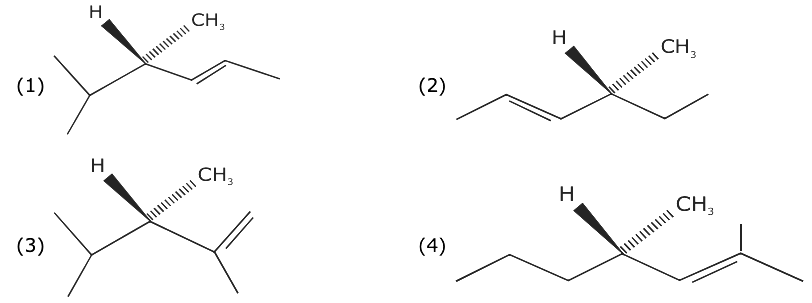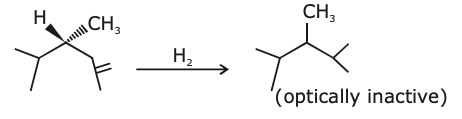Solution: (3)

Question 10: Of the species, NO, NO+, NO2+ and NO, the one with minimum bond strength is:
1) NO
2) NO+
3) NO2+
4) NO

B.O. NO = 2

BO NO+ = 3

BO NO2+ = 2.5

BO NO = 2.5

B.O ∝ 1 / [B.L]

Solution: (1)

Question 11: Glycerol is separated in soap industries by:
1) Fractional distillation
2) Distillation under reduced pressure
3) Differential extraction
4) Steam distillation

conceptual

Glycerol is separated in soap industries by distillation under reduced pressure.

Solution: (2)

Question 12: Thermal power plants can lead to:
1) Ozone layer depletion
2) Blue baby syndrome
3) Eutrophication
4) Acid rain

Refer environmental chemistry

It emits CO2 that combines with moisture of atmosphere and forms H2CO3 (carbonic acid).

Solution: (4)

Question 13: Henry’s constant (in kbar) for four gases ɑ, β, 𝛾, 𝝳 and 𝝳 in water at 298 K is given below:

 ɑ β 𝛾 𝝳 KH 50 2 2 x 10-5 0.5

(density of water = 103 kg m-3 at 298 K)

This table implies that:

1) solubility of at 308 K is lower than at 298 K
2) The pressure of a 55.5 molal solution of is 250 bar
3) has the highest solubility in water at a given pressure
4) The pressure of a 55.5 molal solution of is 1 bar

p = KHX mol fraction of gas in liquid.

On increasing tamp, ‘K’H increases.

Hence solubility decreases.

Solution: (1)

Question 14: The electronic spectrum of [Ti(H2O)6]3+ shows a single broad peak with a maximum at 20,300 cm-1. The crystal field stabilization energy (CFSE) of the complex ion, in kJ mol-1, is:

(1 kJ mol-1 = 83.7 cm-1)

1) 83.7
2) 242.5
3) 145.5
4) 97

[Ti(H2O)6]3+ Ti3+ 3d1 in the octahedral field of ligand.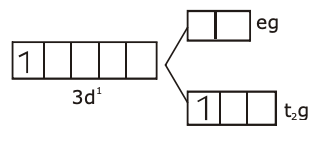CFSE = –0.4 Δ0

CFSE = [0.4 * 20300] / 83.7

= 97 kJ mol

Solution: (4)

Question 15: The atomic number of the element unnilennium is:
1) 109
2) 102
3) 119
4) 108

Solution: (1)

Unnilennium 109

Question 16: An organic compound [A], molecular formula C10H20O2 was hydrolyzed with dilute sulphuric acid to give a carboxylic acid [B] and an alcohol [C]. Oxidation of [C] with CrO3 – H2SO4 produced [B]. Which of the following structures are not possible for [A]?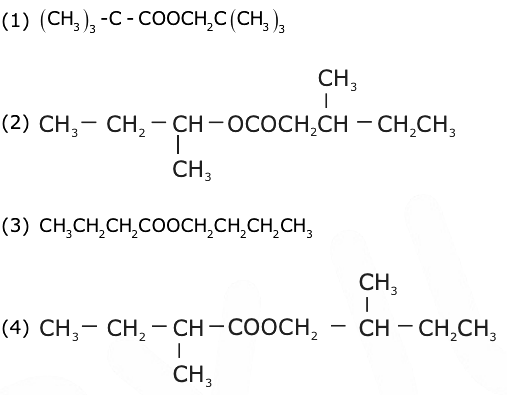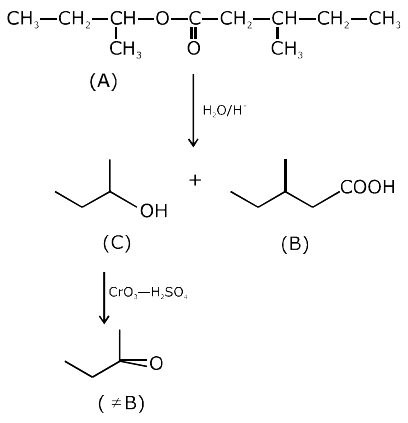Solution: (2)

Question 17: The mechanism of SN1 reaction is given as: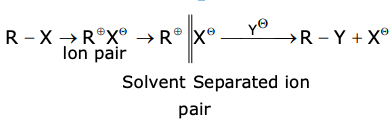A student writes general characteristics based on the given mechanism as:

(a) The reaction is favoured by weak nucleophiles.

(b) R𝝝 would be easily formed if the substituents are bulky.

(c) The reaction is accompanied by racemization.

(d) The reaction is favoured by non-polar solvents. Which observations are correct?

1) (a) and (b)
2) (a), (b) and (c)
3) (a) and (c)
4) (b) and (d)

Solution: (2)

Statement (a), (b) & (c) are correct for SN1 reaction mechanism.

Question 18: Tyndall effect is observed when:
1) The diameter of dispersed particles is much smaller than the wavelength of light used.
2) The diameter of dispersed particles is much larger than the wavelength of light used.
3) The refractive index of the dispersed phase is greater than that of the dispersion medium.
4) The diameter of dispersed particles is similar to the wavelength of light used.

Diameter of dispersed particles should not be much smaller than the wavelength of light used.

Refer topic surface chemistry

Solution: (4)

Question 19: Let CNaCl and CBaSO4 be the conductances (in S) measured for saturated aqueous solutions of NaCl and BaSO4, respectively, at a temperature T. Which of the following is false?
1) CNaCl (T2) > CBaSo4 (T1) for T2 > T1
2) CBaSo4 (T2) > CNaCl (T1) for T2 > T1
3) Ionic mobilities of ions from both salts increase with T.
4) CNaCl >> CBaSo4 at a given T

Ionic

CNaCl >> CBaSo4 at temp ‘T’.

Solution: (4)

Question 20: In a molecule of pyrophosphoric acid, the number of P-OH, P = O and P – O – P bonds / moiety(ies) respectively are:
1) 3, 3 and 3
2) 4, 2 and 1
3) 2, 4 and 1
4) 4, 2 and 0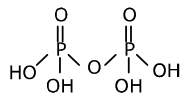P – OH bonds = 4

P = O bonds = 2

P – O – P linkage = 1

4, 2, 1 is the answer.

Solution: (2)

Question 21: The mole fraction of glucose (C6H12O6) in an aqueous binary solution is 0.1. The mass percentage of water in it, to the nearest integer, is _______.

Sol. 47 %

xGlucose = 0.1

mass% of glucose = {[0.1* 180] / [0.1 * 180 + 0.9 * 18]} * 100

= 1800 / [18 + 16.2]

= [1800 / 34.2] %

= 52.63%

= 53%

The mass % of H2O = 47%

Question 22: The volume strength of 8.9 M H2O2 solution calculated at 273 K and 1 atm is ______. (R = 0.0821 L atm K-1 mol-1) (rounded off to the nearest integer)

Volume strength = [8.9 / 2] * [0.821 * 273] / 1

= 99.73

= 100

Solution: 100

Question 23: An element with molar mass 2.7 10-2 kg mol-1 forms a cubic unit cell with edge length 405 pm. If its density is 2.7 103 kg m-3, the radius of the element is approximately ______ 10-12 m (to the nearest integer).

Density = [Z * GMM] / [NA * a3]

2.7 × 103 = [Z * 2.7 * 10-2] / [6.023 * 1023 * (405 * 10-12)]3

Z = 6.023 × 405 × 405 × 405 × 1023–36+3+2

Z = 6.023 × 405 × 405 × 405 × 10–8

Z = 4

FCC

4R = √2 a

R = [405 / 2√2] * 10-12 = 143.21 × 10–12 m

= 143

Solution: 143

Question 24: The total number of monohalogenated organic products in the following (including stereoisomers) reaction is ______.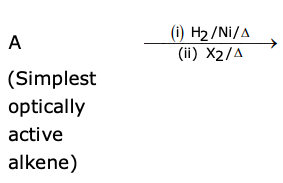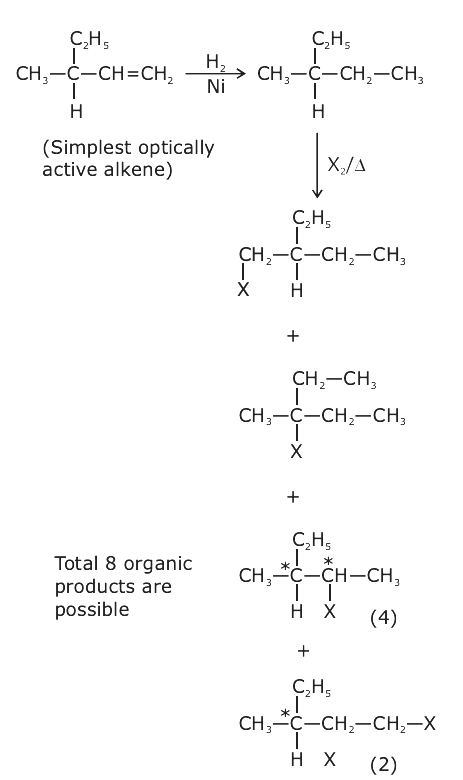Solution: 8

Question 25: The photoelectric current from Na (Work function, w0 = 2.3 eV) is stopped by the output voltage of the cell Pt(s) H2 (g, 1 Bar) HCl (aq. pH =1) AgCl s Ag(s). The pH of aq. HCl required to stop the photoelectric current form K(w0 = 2.25 eV), all other conditions remaining the same, is _______ 10-2 (to the nearest integer). Given,

2.303 (RT / F) = 0.06 V; E0AgCl|Ag|Cl- = 0.22 V

Energy of photon = 2.3 – Ecell {for Na}

Energy of photon = 2.25 – Ecell {for K}

Ecell {for ‘Na’} + 0.05 = Ecell {for ‘K’}

0.22 + 0.06 log [H+][Cl] + 0.05 = 0.22 + 0.06 log [H+] [Cl]

6 log (10–2) + 5 = 6 log [H+][Cl]

log (10–12) + log (105) = log {[H+][Cl]}6

{[H+][Cl]}6 = 10–7

[H+]12 = 10–7

pH = 7 / 12 = 0.58

= 58 × 10–2 = 58

Solution: 58

## Video Lessons – September 3 Shift 1 Chemistry